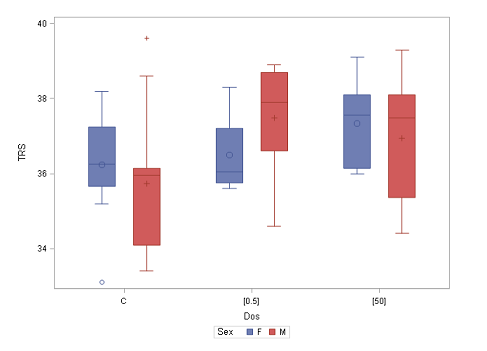3
Oct

–

28
Oct
Uppsala

# Statistics I: Basic statistics, 4 hpThe Ph.D. course will be given in Uppsala 3- 28 October 2022.

Prerequisites: None

## Objective:

The objective of the course is to give an introduction to basic probability theory and statistical inference. On completion of the course, the student will be able to:

• apply basic probability concepts and statistical principles and formulate a problem in statistical terms
• describe common statistical methods including conditions and assumptions
• select an appropriate statistical method for a given problem
• carry out a basic statistical analysis in the statistical software R
• interpret and evaluate results correctly and draw reasonable conclusions
• clearly and concisely communicate results and conclusions

## Content

The course is built on lectures and computer exercises. The main components are as follows:

• Descriptive statistics.
• Basic probability theory.
• Probability distributions.
• Statistical inference of means and proportions, one and two samples.
• Nonparametric methods.
• Analysis of frequency data.

## Facts

Time: 2022-10-03 - 2022-10-28
City: Uppsala
Last signup date: 9 September 2022